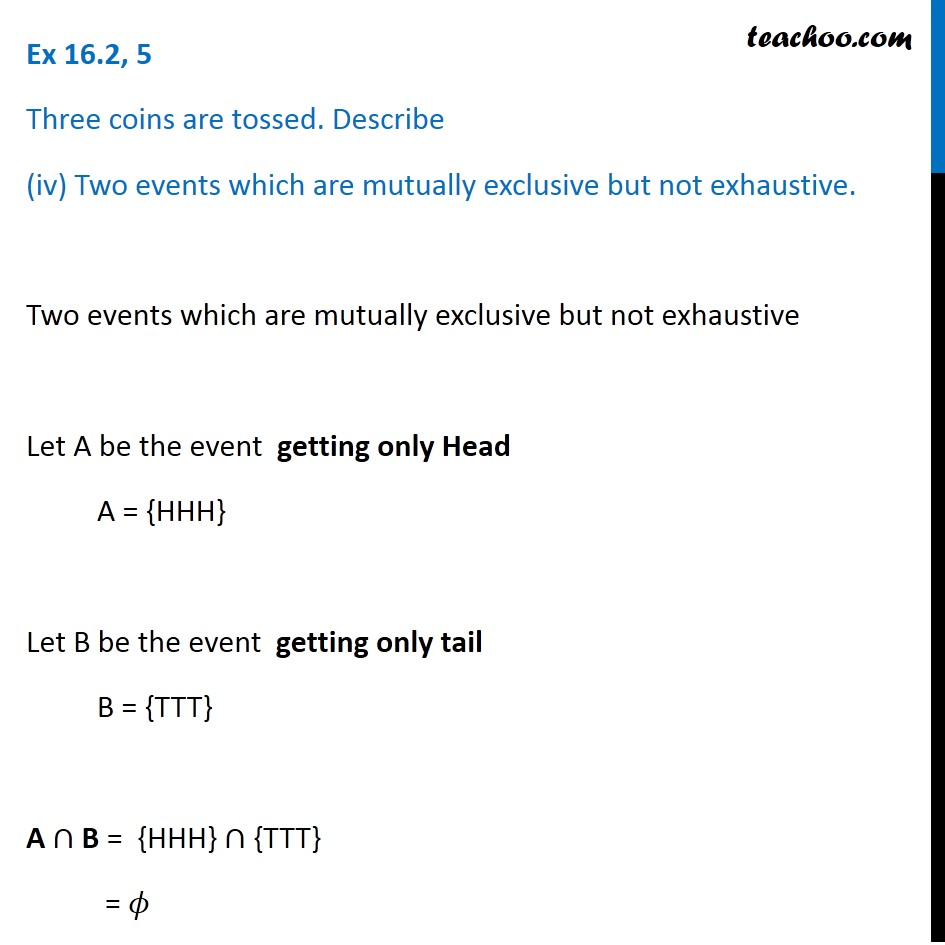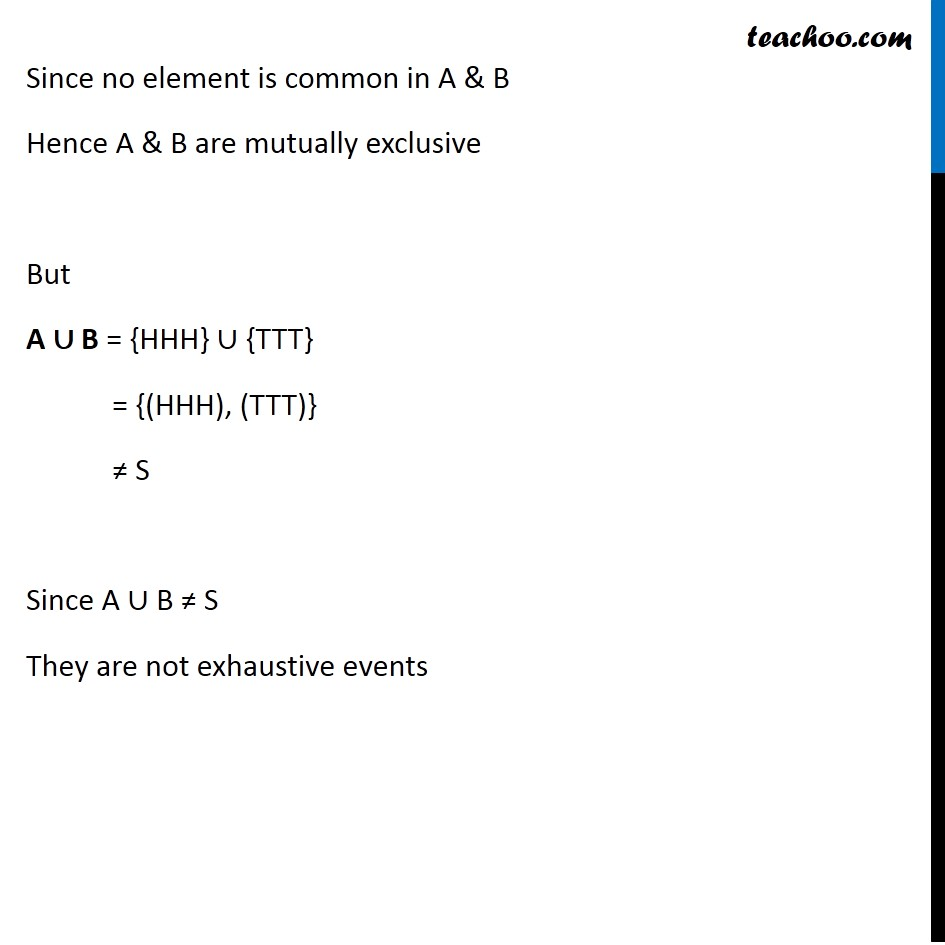1. Chapter 16 Class 11 Probability (Term 2)
2. Serial order wise
3. Ex 16.2

Transcript

Ex 16.2, 5 Three coins are tossed. Describe (iv) Two events which are mutually exclusive but not exhaustive. Two events which are mutually exclusive but not exhaustive Let A be the event getting only Head A = {HHH} Let B be the event getting only tail B = {TTT} A ∩ B = {HHH} ∩ {TTT} = 𝜙 Since no element is common in A & B Hence A & B are mutually exclusive But A ∪ B = {HHH} ∪ {TTT} = {(HHH), (TTT)} ≠ S Since A ∪ B ≠ S They are not exhaustive events